$\newcommand{\dif}{\mathop{}\!\mathrm{d}}$

# 能带与能隙

## 布拉格定律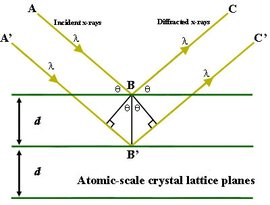## 近自由电子模型（定性分析）$k = \pm \frac{n\pi}{a}, \;\text{(n 为正整数)}$

$\psi(+) = e^{i \frac{\pi}{a}x} + e^{-i\frac{\pi}{a}x} = 2 \cos (\frac{\pi}{a}x)\\ \psi(-) = e^{i \frac{\pi}{a}x} - e^{-i\frac{\pi}{a}x} = 2 \sin (\frac{\pi}{a}x)$

$\rho(+) = |\psi(+)|^2 \propto \cos^2(\pi x/a)\\ \rho(-) = |\psi(-)|^2 \propto \sin^2(\pi x/a)$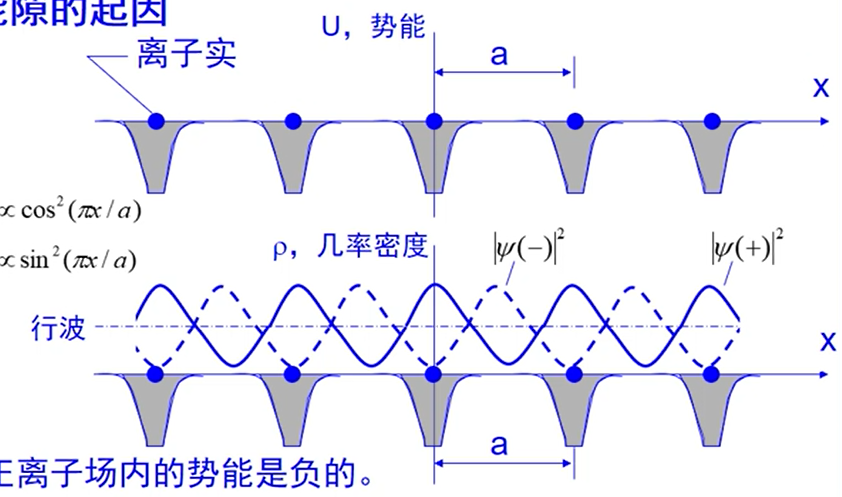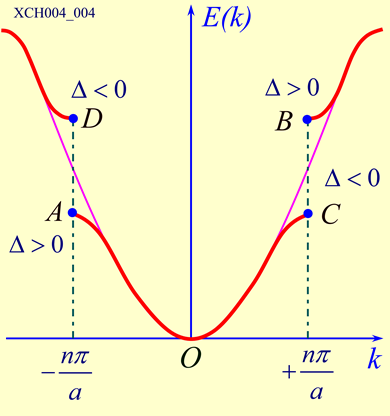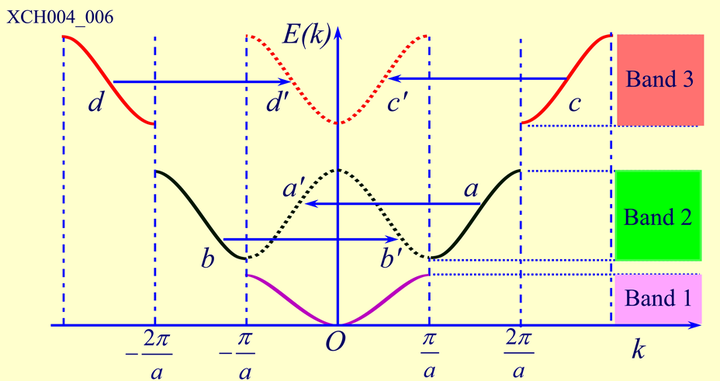• 自由电子模型对应粉线，$\varepsilon_k = \frac{\hbar}{2m}k^2$，是连续的；
• $\psi(+)$ 对应于 AC，即第一允许能带；
• $\psi(-)$ 对应于 DB，即第二允许能带；
• AB之间、CD之间的部分就是能隙（禁带）；

## 布洛赫函数

1. 绝热近似，认为离子实固定在其瞬时位置 上，可把电子的运动与离子实的运动分开来处理；
2. 单电子近似，认为一个电子在离子实和 其它电子所形成的势场中运动；
3. 周期场近似， 假设所有电子及离子实产生的场都具有晶格 周期性。布洛赫电子论相比于金属自由电子论，考虑了电子和离子实之间的相互作用，也考虑了电子与电子的相互作用。

$\psi_\vec{k}(\vec{r}) = u_\vec{k}(\vec{r}) e^{i\vec{k}\cdot \vec{r}}$

## 克朗尼格-朋奈模型（定量分析）

$U(x) = \begin{cases} 0 ,\; (0<x<a)\\ U_0 ,\; (a<x<a+b) \end{cases}$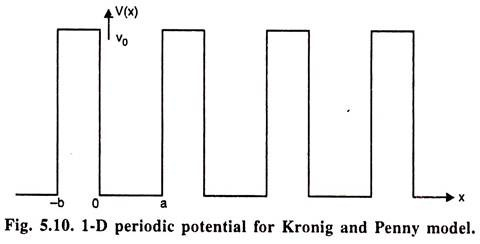$\psi = \begin{cases} A e^{iKx} + B e^{-iKx} ,\; (0<x<a)\\ C e^{Qx} + De^{-Qx} ,\; (0<x<a+b) \end{cases}$

1. $\psi$ 和 $\dif \psi/\dif x$ 在 0 和 a 处连续；
2. $\psi$ 和 $\dif \psi/\dif x$ 在 a 处的值等于 -b 处的值，但超前一个相位因子 $\exp [ik(a+b)]$（布洛赫定理）。

$(P/Ka) \sin Ka + \cos Ka = \cos ka$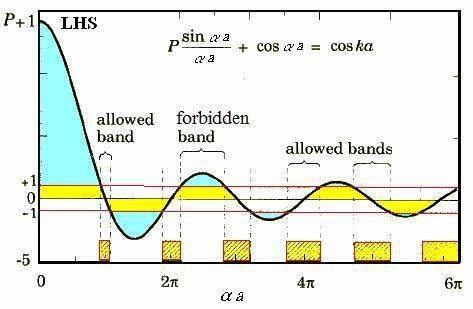$\varepsilon = \begin{cases} \frac{\hbar^2 K^2}{2m} ,\; (0<x<a)\\ U_0 - \frac{\hbar^2 Q^2}{2m} ,\; (a<x<a+b) \end{cases}$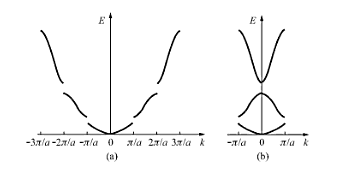## 半导体晶体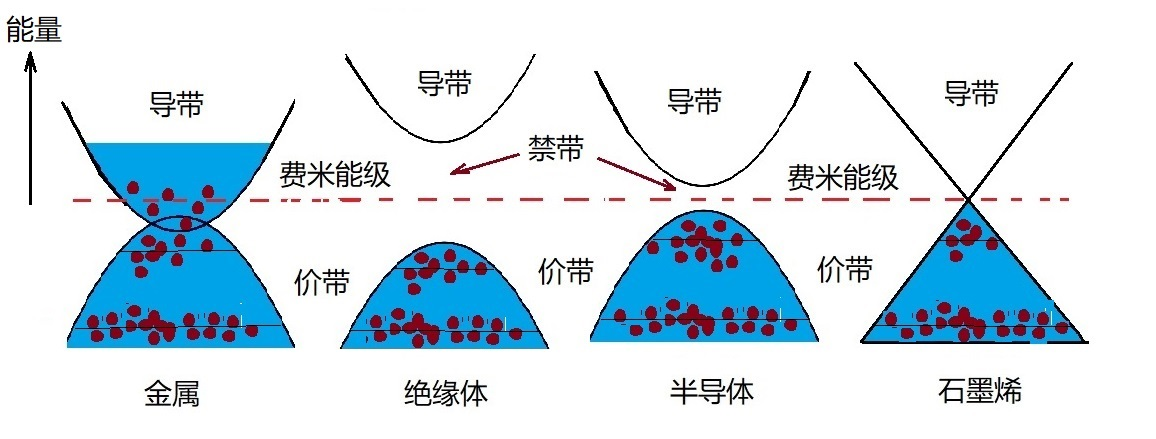• 直接能隙晶体：导带底和价带顶的 K 相同，当电子吸光跃迁时，不需要动量，非常容易吸收或发出光子。比如：GaAs，常用来作激光。
• 间接能隙晶体：导带底和价带顶的 K 不相同，跃迁除了需要光子，还需要声子（晶格的振动），以满足动量守恒。比如 Si，但仍能用作太阳能。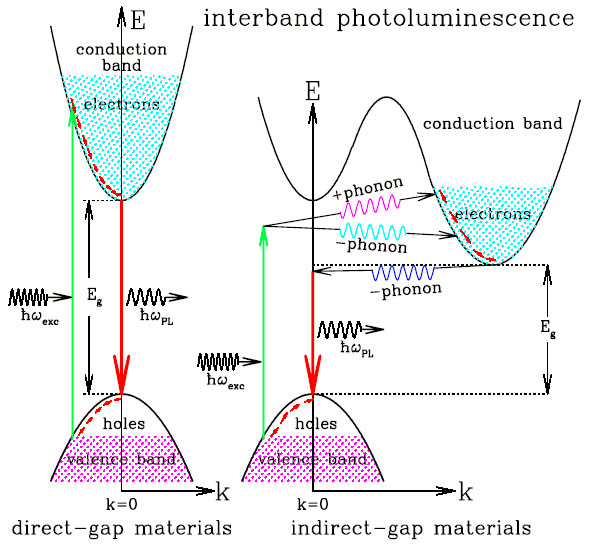## 硅的能带